Next: Origin of Permanent Magnetism Up: Magnetism Previous: Ampère's Circuital Law

## Magnetic Field of a Solenoid

A solenoid is a tightly wound helical coil of wire whose diameter is small compared to its length. The magnetic field generated in the centre, or core, of a current carrying solenoid is essentially uniform, and is directed along the axis of the solenoid. Outside the solenoid, the magnetic field is far weaker. Figure 27 shows (rather schematically) the magnetic field generated by a typical solenoid. The solenoid is wound from a single helical wire which carries a current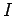. The winding is sufficiently tight that each turn of the solenoid is well approximated as a circular wire loop, lying in the plane perpendicular to the axis of the solenoid, which carries a current. Suppose that there are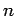such turns per unit axial length of the solenoid. What is the magnitude of the magnetic field in the core of the solenoid?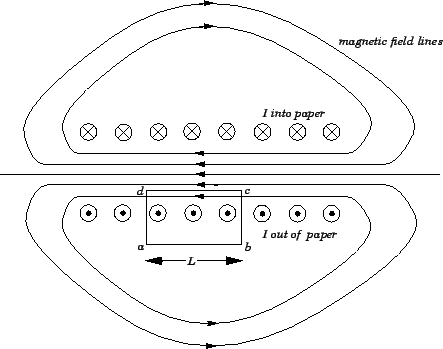In order to answer this question, let us apply Ampère's circuital law to the rectangular loop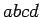. We must first find the line integral of the magnetic field around. Along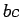and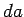the magnetic field is essentially perpendicular to the loop, so there is no contribution to the line integral from these sections of the loop. Alongthe magnetic field is approximately uniform, of magnitude, say, and is directed parallel to the loop. Thus, the contribution to the line integral from this section of the loop is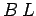, where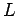is the length of. Along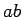the magnetic field-strength is essentially negligible, so this section of the loop makes no contribution to the line integral. It follows that the line integral of the magnetic field aroundis simply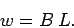(178)

By Ampère's circuital law, this line integral is equal to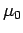times the algebraic sum of the currents which flow through the loop. Since the length of the loop along the axis of the solenoid is, the loop intersects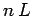turns of the solenoid, each carrying a current. Thus, the total current which flows through the loop is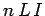. This current counts as a positive current since if we look against the direction of the currents flowing in each turn (i.e., into the page in the figure), the loopcirculates these currents in an anti-clockwise direction. Ampère's circuital law yields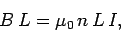(179)

which reduces to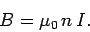(180)

Thus, the magnetic field in the core of a solenoid is directly proportional to the product of the current flowing around the solenoid and the number of turns per unit length of the solenoid. This, result is exact in the limit in which the length of the solenoid is very much greater than its diameter.Next: Origin of Permanent Magnetism Up: Magnetism Previous: Ampère's Circuital Law
Richard Fitzpatrick 2007-07-14